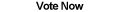Home Articles Components Code Snippets Links# C# Pick Random Elements Based on Probability

## C# Random Elements

Simple problem: I have a list of elements and I want to choose one randomly based on the probability of each element.

The nature of the "randomness" is not important here, more important is the probabiliy that each element (in an array for example) is selected compared to the other elements. For the random aspect, check out the article on generating actually random numbers in C#.

## Example

So let's say we have four elements, and each has a 25% chance of being chosen:

```A - 25%
B - 25%
C - 25%
D - 25%          ```

How do we randomly pick one? In .NET it is easy, using the Random class we can generate a pseudo-random number between 0 and 3 (index) and that will be the element we randomly selected. This works because the chance of picking one of the four elements is the same for all of them.

But now let's modify the list:

```A - 35%
B - 15%
C - 40%
D - 10%```

We can't just randomly pick an index anymore.

## Statistics

The answer is pretty simple with some basic statistics knowledge. Instead of randomly selecting an index, we generate a random number between 0 and 100 (or 0.0 and 1.0) and compare that to the cumulative probability of each element. The cumulative probability is simply the probability of the element plus the probability of all the elements before it.

Let's apply that to our example, first let's sort the elements:

```Element - Probability - Cumulative Probability
D          10%               10%
B          15%               25%
A          35%               60%
C          40%               100%```

Now let's roll the die... let's say we get 53. We compare that number to each element's cumulative probability and select the first one that is within range. So D is 10%, not within 53. Next is B, 25%, no good. Next is A, 60%, that is greater than 53! Thus the element randomly chosen with our random number 53 is A.

This makes sense because elements with low probability will need a small random number so they can be chosen right off the bat. Elements with higher probability will "leverage" the probability of the smaller ones to have a better chance of getting chosen.

## C# Code

This is a coding website, so let's write down some simple code:

```List<KeyValuePair<string, double>> elements = ...some data
Random r = new Random();
double diceRoll = r.NextDouble();

double cumulative = 0.0;
for (int i = 0; i < elements.Count; i++)
{
cumulative += elements[i].Value;
if (diceRoll < cumulative)
{
string selectedElement = elements[i].Key;
break;
}
}
```

In this case, each element is a keyvalue pair, with the key being the element name and the value being the probability of that element (between 0.0 and 1.0).

One final note. It is pretty obvious, but make sure the probabiliy of all the elements add up to 1 (or 100 depending on your range). Simple fact, but can cause some headaches if you forget.

Back to C# Article List